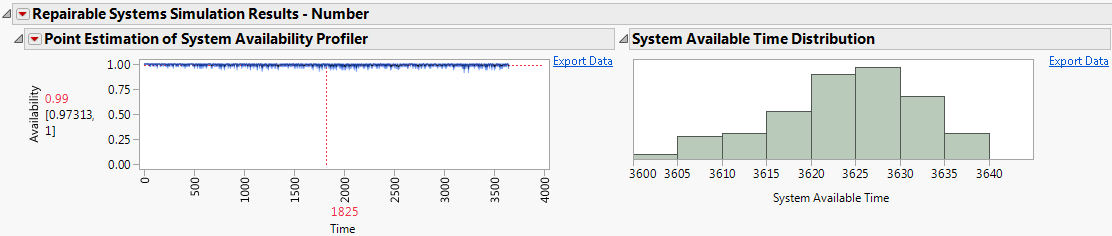Publication date: 11/10/2021

##Results Explorer

When you click the green arrow next to the Launch Repairable Systems Simulation Results Explorer script in the results data table, a report appears that contains an analysis of the simulation results.

Figure 12.11 Partial RSS Explorer ReportBy default, the report contains two types of graphs. The first type of graph, shown on the left side of Figure 12.11, is a point estimate graph. The point estimate graph displays aggregated simulation results on the vertical axis plotted against simulation iteration time on the horizontal axis. The range of the horizontal axis is the duration that you specify in the simulation settings. The estimated probability of the system being available at a given time is shown in red next to the vertical axis. Below the point estimate is a 95% confidence interval for the point estimate.

Tip: To see the point estimation of system availability at a specific time in the simulation iteration, click the red number below the horizontal axis. Specify a time within the range of the simulation duration and press Enter.

The second type of graph, shown on the right side of Figure 12.11, is a histogram of the system available time from the simulation iterations. The bins in the histograms are representative of one of the following:

Total simulation time.

Proportion of the simulation duration.

Tip: Select the Export Data option beside a graph to create a data table with the data that the graph uses.

By default, the report contains the following graphs:

Point Estimation of System Availability Profiler

Shows a profiler graph over time of the estimated probability that the system is in the On or the Off state during a simulation iteration.

System Available Time Distribution

Shows a histogram of the total time that the system was available for each simulation iteration.

System Availability Distribution

Shows a histogram of the total time that the system was available for each simulation iteration divided by the duration of the simulation. This distribution is the same as the System Available Time Distribution, except that it is shown as a proportion of the duration of the simulation.

Point Estimation of System In Service Probability Profiler

Shows a profiler graph over time of the estimated probability that the system is in the On state during a simulation iteration.

System In Service Time Distribution

Shows a histogram of the total time that the system was in the On state for each simulation iteration.

System In Service Probability Distribution

Shows a histogram of the total time that the system was in the On state for each simulation iteration divided by the duration of the simulation. This distribution is the same as System In Service Time Distribution, except that the distribution is shown as a proportion of the duration of the simulation.

Point Estimation of System Unplanned Outage Profiler

Shows a profiler graph over time of the estimated probability that the system is in the Down state during a simulation iteration.

System Unplanned Outage Distribution

Shows a histogram of the total time that the system was in the Down state for each simulation iteration.

System Unplanned Outage Percentage Distribution

Shows a histogram of the total time that the system was in the Down state for each simulation iteration divided by the duration of the simulation. This distribution is the same as System Unplanned Outage Distribution, except that the distribution is shown as a proportion of the duration of the simulation.